Have a question about one of the blog posts? Chat here with me.

# Maps with pie charts on top of each administrative division: an example with Luxembourg's elections data

R

## Abstract

You can find the data used in this blog post here: https://github.com/b-rodrigues/elections_lux

This is a follow up to a previous blog post where I extracted data of the 2018 Luxembourguish elections from Excel Workbooks. Now that I have the data, I will create a map of Luxembourg by commune, with pie charts of the results on top of each commune! To do this, I use good ol’ `{ggplot2}` and another packages called `{scatterpie}`. As a bonus, I have added the code to extract the data from the 2013 elections from Excel. You’ll find this code in the appendix at the end of the blog post.

## Introduction

Before importing the data for the elections of 2018, let’s install some packages:

``````install.packages('rgeos', type='source') # Dependency of rgdal
install.packages('rgdal', type='source') # To read in the shapefile``````

These packages might be very tricky to install on OSX and Linux, but they’re needed to import the shapefile of the country, which is needed to draw a map. So to make things easier, I have created an `rds` object, from the shapefile of Luxembourg, that you can import natively in R without needing these two packages. But if you want to use them, here is how:

``communes <- readOGR("Limadmin_SHP/LIMADM_COMMUNES.shp")``

I’ll use my shapefile though (that you can download from the same github repo as the data):

``communes_df <- readRDS("commune_shapefile.rds")``

Here’s how it looks like:

``head(communes_df)``
``````##       long      lat order  hole piece      group       id
## 1 91057.65 101536.6     1 FALSE     1 Beaufort.1 Beaufort
## 2 91051.79 101487.3     2 FALSE     1 Beaufort.1 Beaufort
## 3 91043.43 101461.7     3 FALSE     1 Beaufort.1 Beaufort
## 4 91043.37 101449.8     4 FALSE     1 Beaufort.1 Beaufort
## 5 91040.42 101432.1     5 FALSE     1 Beaufort.1 Beaufort
## 6 91035.44 101405.6     6 FALSE     1 Beaufort.1 Beaufort``````

``````library("tidyverse")
library("tidyxl")
library("ggplot2")
library("scatterpie")``````

Ok, now, let’s import the elections results data, which is the output of last week’s blog post:

``elections <- read_csv("elections_2018.csv")``
``````## Parsed with column specification:
## cols(
##   Party = col_character(),
##   Year = col_double(),
##   Variables = col_character(),
##   Values = col_double(),
##   locality = col_character(),
##   division = col_character()
## )``````

I will only focus on the data at the commune level, and only use the share of votes for each party:

``````elections_map <- elections %>%
filter(division == "Commune",
Variables == "Pourcentage")``````

Now I need to make sure that the names of the communes are the same between the elections data and the shapefile. Usual suspects are the “Haute-Sûre” and the “Redange-sur-Attert” communes, but let’s take a look:

``````locality_elections <- unique(elections_map\$locality)
locality_shapefile <- unique(communes_df\$id)

setdiff(locality_elections, locality_shapefile)``````
``##  "Lac de la Haute-Sûre" "Redange Attert"``

Yep, exactly as expected. I’ve had problems with the names of these two communes in the past already. Let’s rename these two communes in the elections data:

``````elections_map <- elections_map %>%
mutate(commune = case_when(locality == "Lac de la Haute-Sûre" ~ "Lac de la Haute Sûre",
locality == "Redange Attert" ~ "Redange",
TRUE ~ locality))``````

Now, I can select the relevant columns from the shapefile:

``````communes_df <- communes_df %>%
select(long, lat, commune = id)``````

and from the elections data:

``````elections_map <- elections_map %>%
select(commune, Party, Variables, Values)``````

## Plotting the data on a map

Now, for the type of plot I want to make, using the `{scatterpie}` package, I need the data to be in the wide format, not long. For this I will use `tidyr::spread()`:

``````elections_map <- elections_map %>%

This is how the data looks now:

``glimpse(elections_map)``
``````## Observations: 102
## Variables: 10
## \$ commune     <chr> "Beaufort", "Bech", "Beckerich", "Berdorf", "Bertran…
## \$ Variables   <chr> "Pourcentage", "Pourcentage", "Pourcentage", "Pource…
## \$ ADR         <dbl> 0.12835106, 0.09848661, 0.08596748, 0.16339234, 0.04…
## \$ CSV         <dbl> 0.2426239, 0.2945285, 0.3004751, 0.2604552, 0.290278…
## \$ `déi gréng` <dbl> 0.15695672, 0.21699651, 0.24072721, 0.15619529, 0.15…
## \$ `déi Lénk`  <dbl> 0.04043732, 0.03934808, 0.05435776, 0.02295273, 0.04…
## \$ DP          <dbl> 0.15875393, 0.19394645, 0.12899689, 0.15444466, 0.30…
## \$ KPL         <dbl> 0.015875393, 0.006519208, 0.004385164, 0.011476366, …
## \$ LSAP        <dbl> 0.11771754, 0.11455180, 0.08852549, 0.16592103, 0.09…
## \$ PIRATEN     <dbl> 0.13928411, 0.03562282, 0.09656496, 0.06516242, 0.04…``````

For this to work, I need two datasets; one to draw the map (`commune_df`) and one to draw the pie charts over each commune, with the data to draw the charts, but also the position of where I want the pie charts. For this, I will compute the average of the longitude and latitude, which should be good enough:

``````scatterpie_data <- communes_df %>%
group_by(commune) %>%
summarise(long = mean(long),
lat = mean(lat))``````

Now, let’s join the two datasets:

``final_data <- left_join(scatterpie_data, elections_map, by = "commune") ``

I have all the ingredients to finally plot the data:

``````ggplot() +
geom_polygon(data = communes_df, aes(x = long, y = lat, group = commune), colour = "grey", fill = NA) +
geom_scatterpie(data = final_data, aes(x=long, y=lat, group=commune),
cols = c("ADR", "CSV", "déi gréng", "déi Lénk", "DP", "KPL", "LSAP", "PIRATEN")) +
labs(title = "Share of total vote in each commune, 2018 elections") +
theme_void() +
theme(legend.position = "bottom",
legend.title = element_blank(),
legend.text = element_text(colour = "white"),
plot.background = element_rect("#272b30"),
plot.title = element_text(colour = "white")) +
"CSV" = "#ee7d00",
"déi gréng" = "#45902c",
"déi Lénk" = "#e94067",
"DP" = "#002a54",
"KPL" = "#ff0000",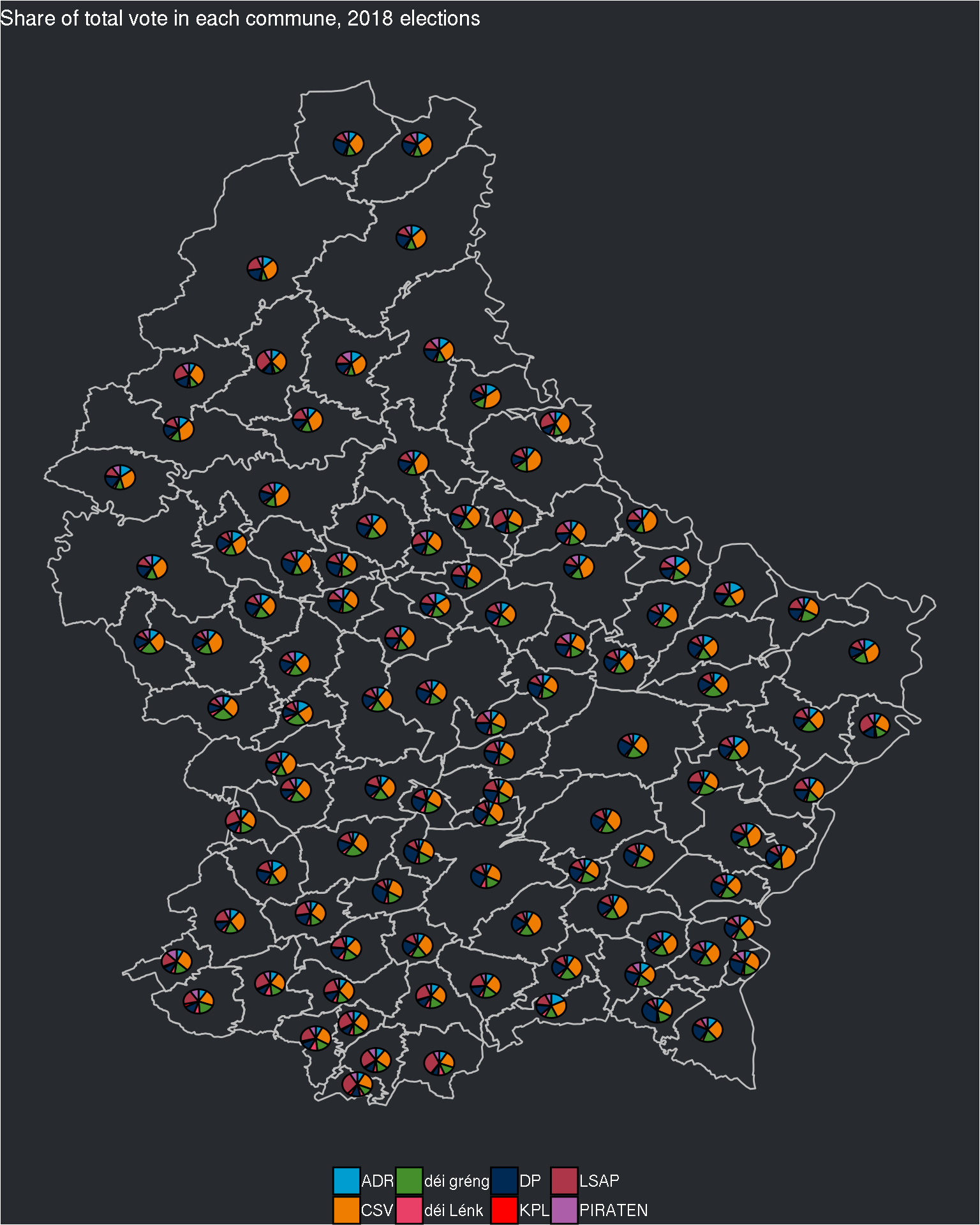Not too bad, but we can’t really read anything from the pie charts. I will now make their size proportional to the number of voters in each commune. For this, I need to go back to the Excel sheets, and look for the right cell: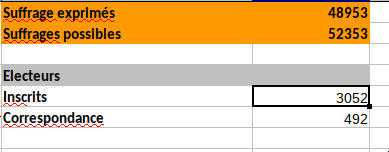It will be easy to extract this info. It located in cell “E5”:

``````elections_raw_2018 <- xlsx_cells("leg-2018-10-14-22-58-09-737.xlsx")

electors_commune <- elections_raw_2018 %>%
filter(!(sheet %in% c("Le Grand-Duché de Luxembourg", "Centre", "Est", "Nord", "Sud", "Sommaire"))) %>%
select(sheet, numeric) %>%
rename(commune = sheet,
electors = numeric)``````

I can now add this to the data:

``````final_data <- final_data %>%
full_join(electors_commune) %>%
mutate(log_electors = log(electors) * 200)``````
``## Joining, by = "commune"``

In the last line, I create a new column called `log_electors` that I then multiply by 200. This will be useful later.

Now I can add the `r` argument inside the `aes()` function on the third line, to make the pie chart size proportional to the number of electors in that commune:

``````ggplot() +
geom_polygon(data = communes_df, aes(x = long, y = lat, group = commune), colour = "grey", fill = NA) +
geom_scatterpie(data = final_data, aes(x=long, y=lat, group = commune, r = electors),
cols = c("ADR", "CSV", "déi gréng", "déi Lénk", "DP", "KPL", "LSAP", "PIRATEN")) +
labs(title = "Share of total vote in each commune, 2018 elections") +
theme_void() +
theme(legend.position = "bottom",
legend.title = element_blank(),
legend.text = element_text(colour = "white"),
plot.background = element_rect("#272b30"),
plot.title = element_text(colour = "white")) +
"CSV" = "#ee7d00",
"déi gréng" = "#45902c",
"déi Lénk" = "#182024",
"DP" = "#002a54",
"KPL" = "#ff0000",
``## Warning: Removed 32 rows containing non-finite values (stat_pie).``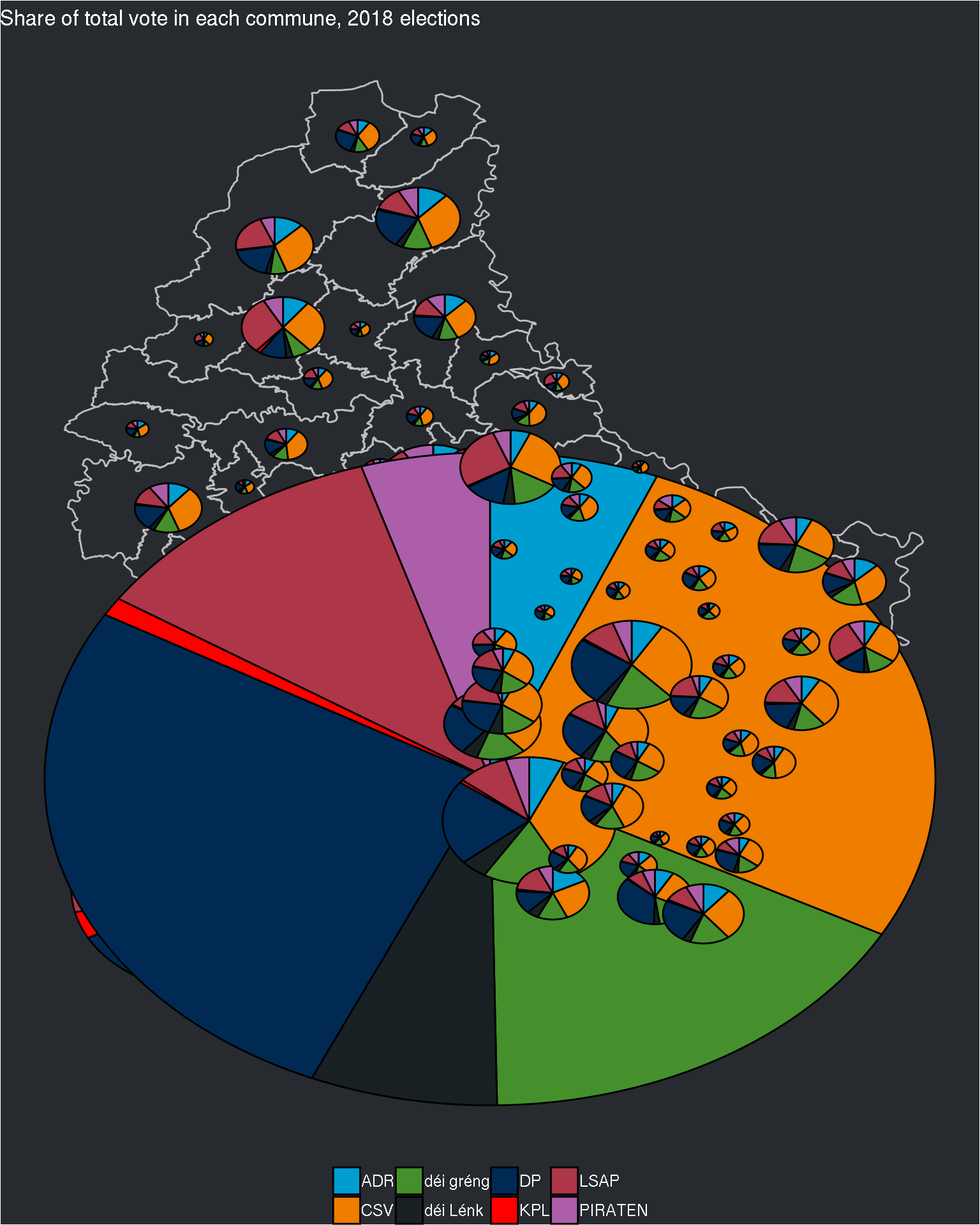Ok, that was not a good idea! Perhaps the best option would be to have one map per circonscription. For this, I need the list of communes by circonscription. This is available on Wikipedia. Here are the lists:

``````centre <- c("Bissen", "Colmar-Berg", "Fischbach", "Heffingen", "Larochette",
"Lintgen", "Lorentzweiler", "Mersch", "Nommern", "Helperknapp", "Bertrange", "Contern",
"Hesperange", "Luxembourg", "Niederanven", "Sandweiler", "Schuttrange", "Steinsel",
"Strassen", "Walferdange", "Weiler-la-Tour")

est <- c("Beaufort", "Bech", "Berdorf", "Consdorf", "Echternach", "Rosport-Mompach", "Waldbillig",
"Betzdorf", "Biwer", "Flaxweiler", "Grevenmacher", "Junglinster", "Manternach", "Mertert",
"Wormeldange","Bous", "Dalheim", "Lenningen", "Mondorf-les-Bains", "Remich", "Schengen",

nord <- c("Clervaux", "Parc Hosingen", "Troisvierges", "Weiswampach", "Wincrange", "Bettendorf",
"Bourscheid", "Diekirch", "Erpeldange-sur-Sûre", "Ettelbruck", "Feulen", "Mertzig", "Reisdorf",
"Schieren", "Vallée de l'Ernz", "Beckerich", "Ell", "Grosbous", "Préizerdaul",
"Rambrouch", "Redange", "Saeul", "Useldange", "Vichten", "Wahl", "Putscheid", "Tandel",
"Vianden", "Boulaide", "Esch-sur-Sûre", "Goesdorf", "Kiischpelt", "Lac de la Haute Sûre",
"Wiltz", "Winseler")

sud <- c("Dippach", "Garnich", "Käerjeng", "Kehlen", "Koerich", "Kopstal", "Mamer",
"Habscht", "Steinfort", "Bettembourg", "Differdange", "Dudelange", "Esch-sur-Alzette",
"Frisange", "Kayl", "Leudelange", "Mondercange", "Pétange", "Reckange-sur-Mess", "Roeser",
"Rumelange", "Sanem", "Schifflange")

circonscriptions <- list("centre" = centre, "est" = est,
"nord" = nord, "sud" = sud)``````

Now, I can make one map per circonscription. First, let’s split the data sets by circonscription:

``````communes_df_by_circonscription <- circonscriptions %>%
map(~filter(communes_df, commune %in% .))

final_data_by_circonscription <- circonscriptions %>%
map(~filter(final_data, commune %in% .))``````

By using `pmap()`, I can reuse the code to generate the plot to each element of the two lists. This is nice because I do not need to copy and paste the code 4 times:

``````pmap(list(x = communes_df_by_circonscription,
y = final_data_by_circonscription,
z = names(communes_df_by_circonscription)),
function(x, y, z){
ggplot() +
geom_polygon(data = x, aes(x = long, y = lat, group = commune),
colour = "grey", fill = NA) +
geom_scatterpie(data = y, aes(x=long, y=lat, group = commune),
cols = c("ADR", "CSV", "déi gréng", "déi Lénk", "DP", "KPL", "LSAP", "PIRATEN")) +
labs(title = paste0("Share of total vote in each commune, 2018 elections for circonscription ", z)) +
theme_void() +
theme(legend.position = "bottom",
legend.title = element_blank(),
legend.text = element_text(colour = "white"),
plot.background = element_rect("#272b30"),
plot.title = element_text(colour = "white")) +
"CSV" = "#ee7d00",
"déi gréng" = "#45902c",
"déi Lénk" = "#182024",
"DP" = "#002a54",
"KPL" = "#ff0000",
}
)``````
``## \$centre``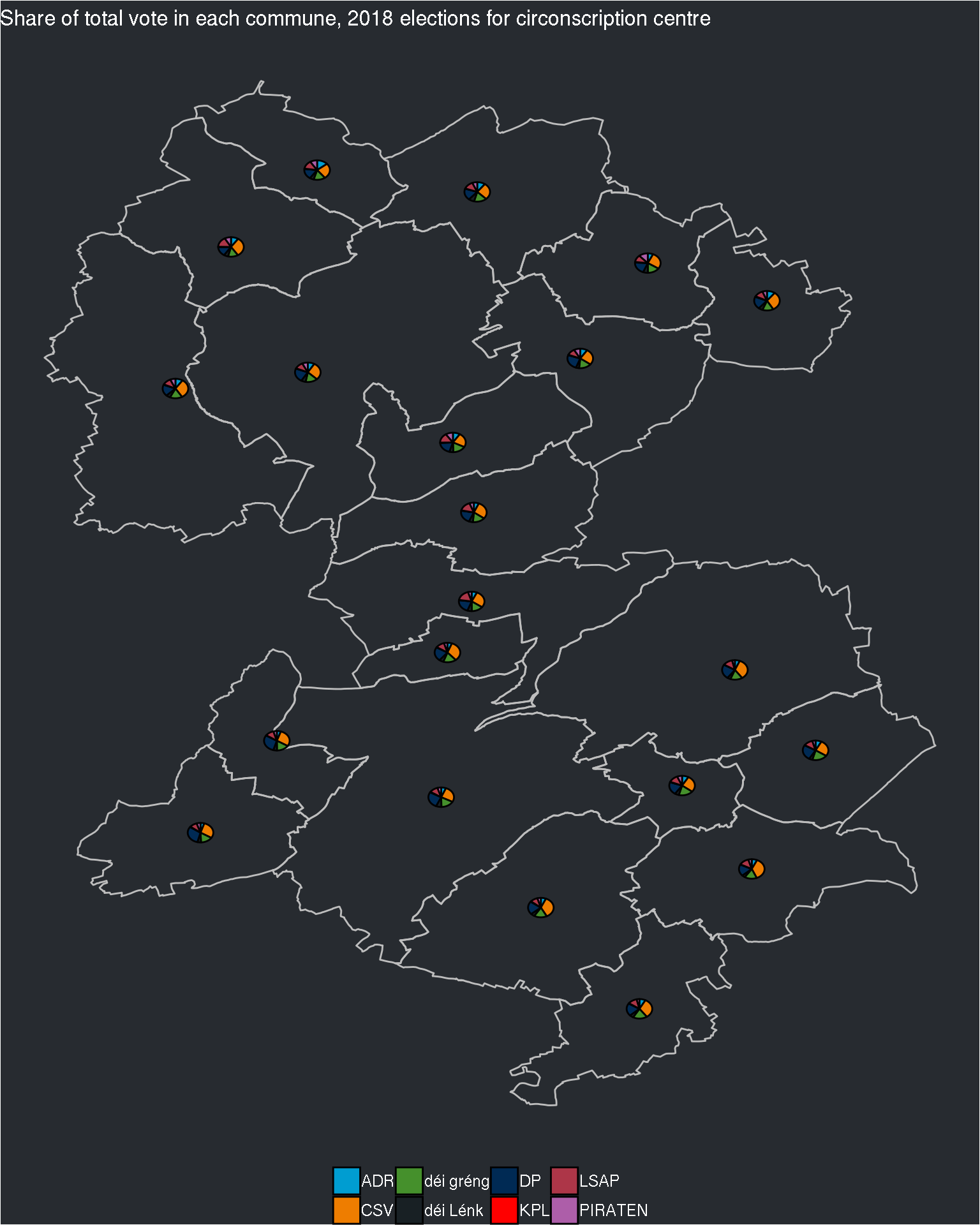``````##
## \$est``````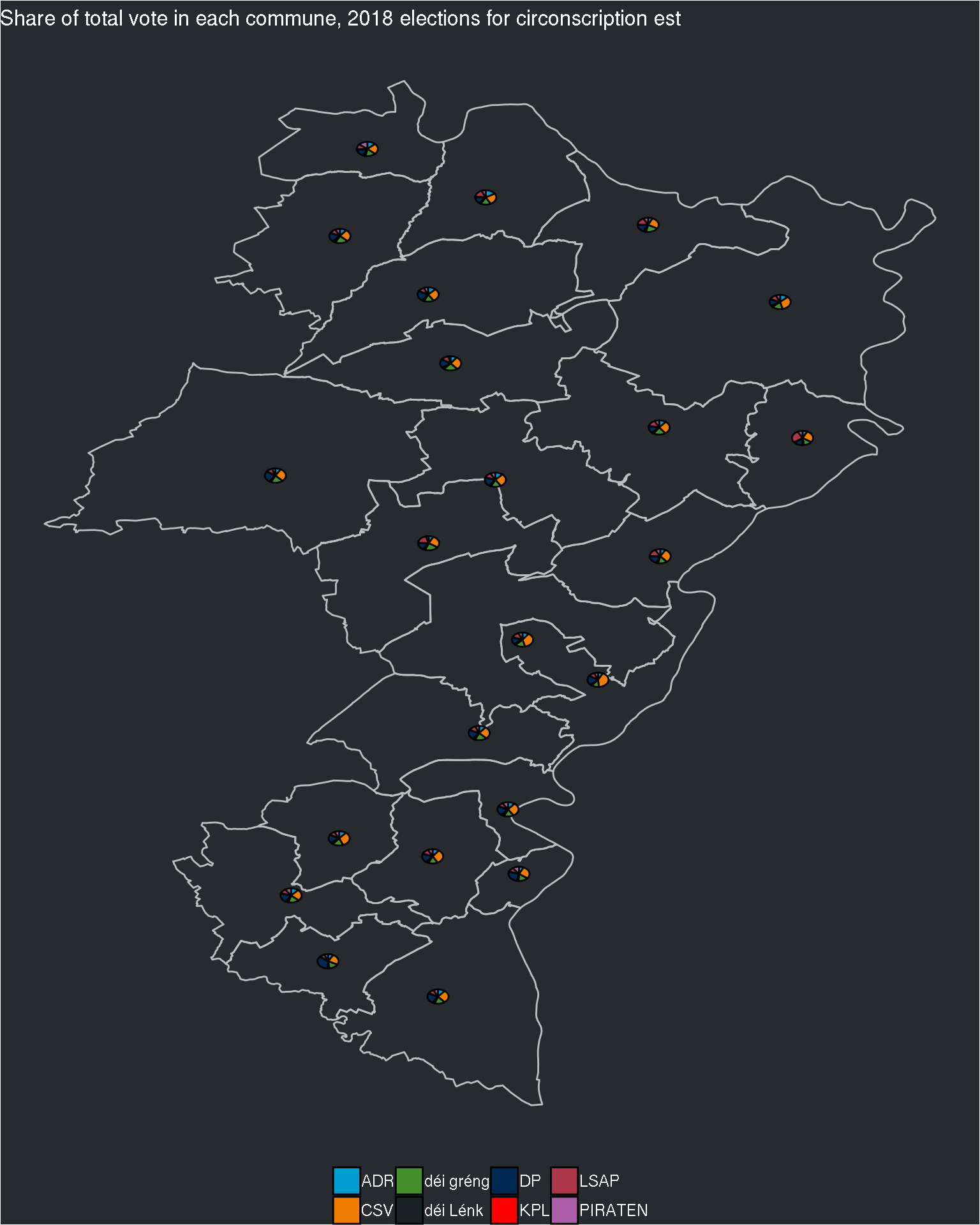``````##
## \$nord``````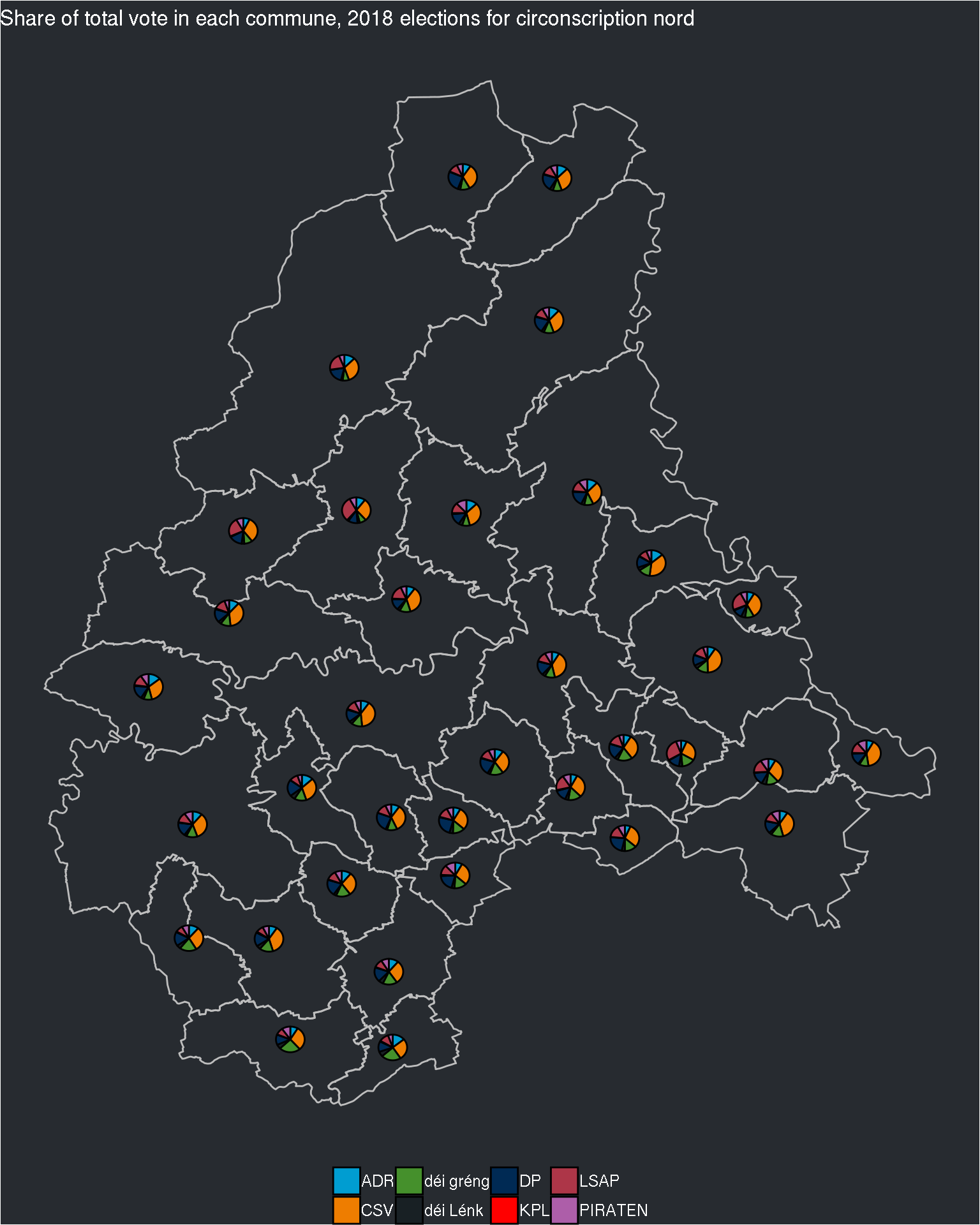``````##
## \$sud``````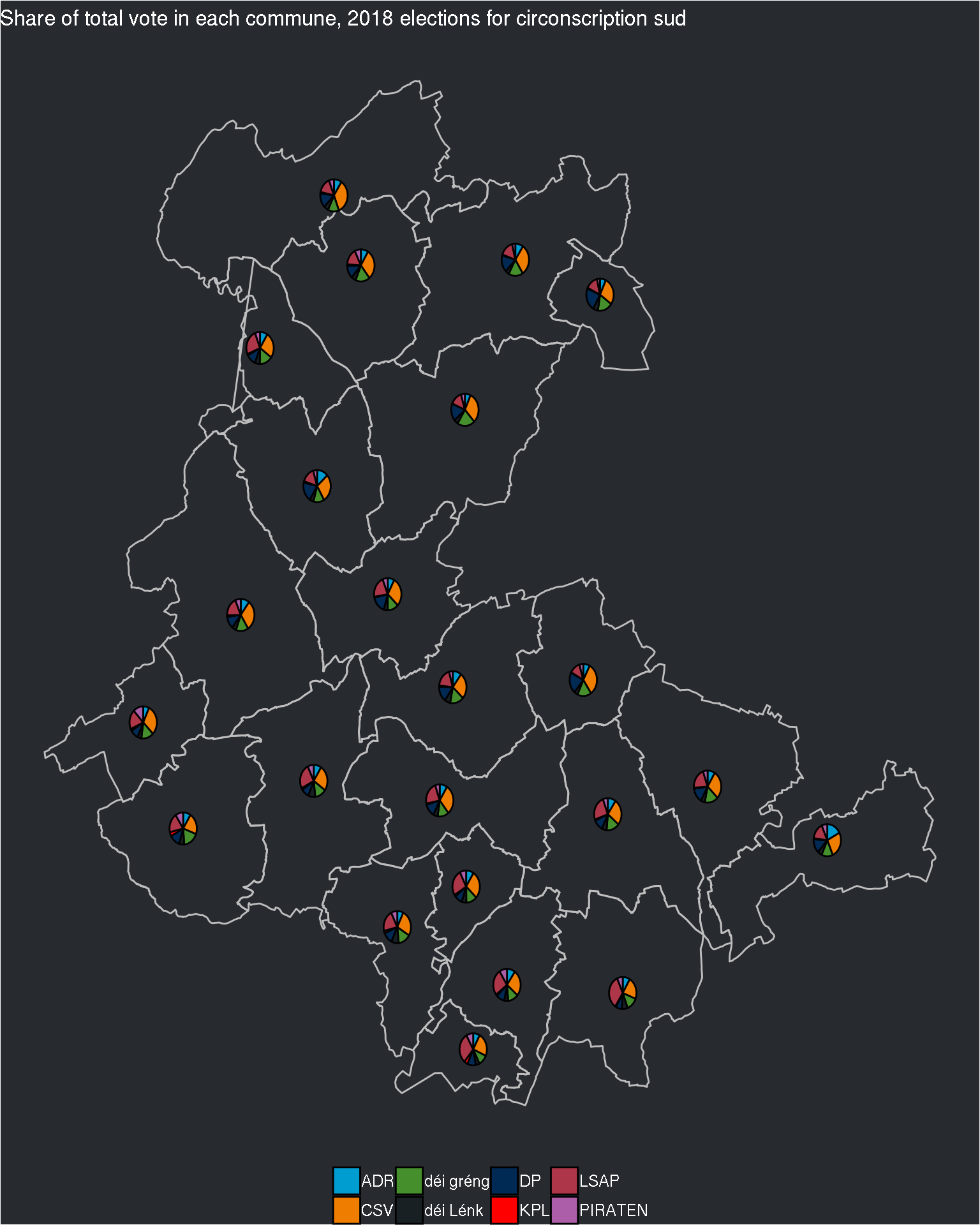I created an anonymous function of three argument, `x`, `y` and `z`. If you are unfamiliar with `pmap()`, study the above code closely. If you have questions, do not hesitate to reach out!

The pie charts are still quite small, but if I try to change the size of the pie charts, I’ll have the same problem as before: inside the same circonscription, some communes have really a lot of electors, and some a very small number. Perhaps I can try with the log of the electors?

``````pmap(list(x = communes_df_by_circonscription,
y = final_data_by_circonscription,
z = names(communes_df_by_circonscription)),
function(x, y, z){
ggplot() +
geom_polygon(data = x, aes(x = long, y = lat, group = commune),
colour = "grey", fill = NA) +
geom_scatterpie(data = y, aes(x=long, y=lat, group = commune, r = log_electors),
cols = c("ADR", "CSV", "déi gréng", "déi Lénk", "DP", "KPL", "LSAP", "PIRATEN")) +
labs(title = paste0("Share of total vote in each commune, 2018 elections for circonscription ", z)) +
theme_void() +
theme(legend.position = "bottom",
legend.title = element_blank(),
legend.text = element_text(colour = "white"),
plot.background = element_rect("#272b30"),
plot.title = element_text(colour = "white")) +
"CSV" = "#ee7d00",
"déi gréng" = "#45902c",
"déi Lénk" = "#182024",
"DP" = "#002a54",
"KPL" = "#ff0000",
}
)``````
``## \$centre``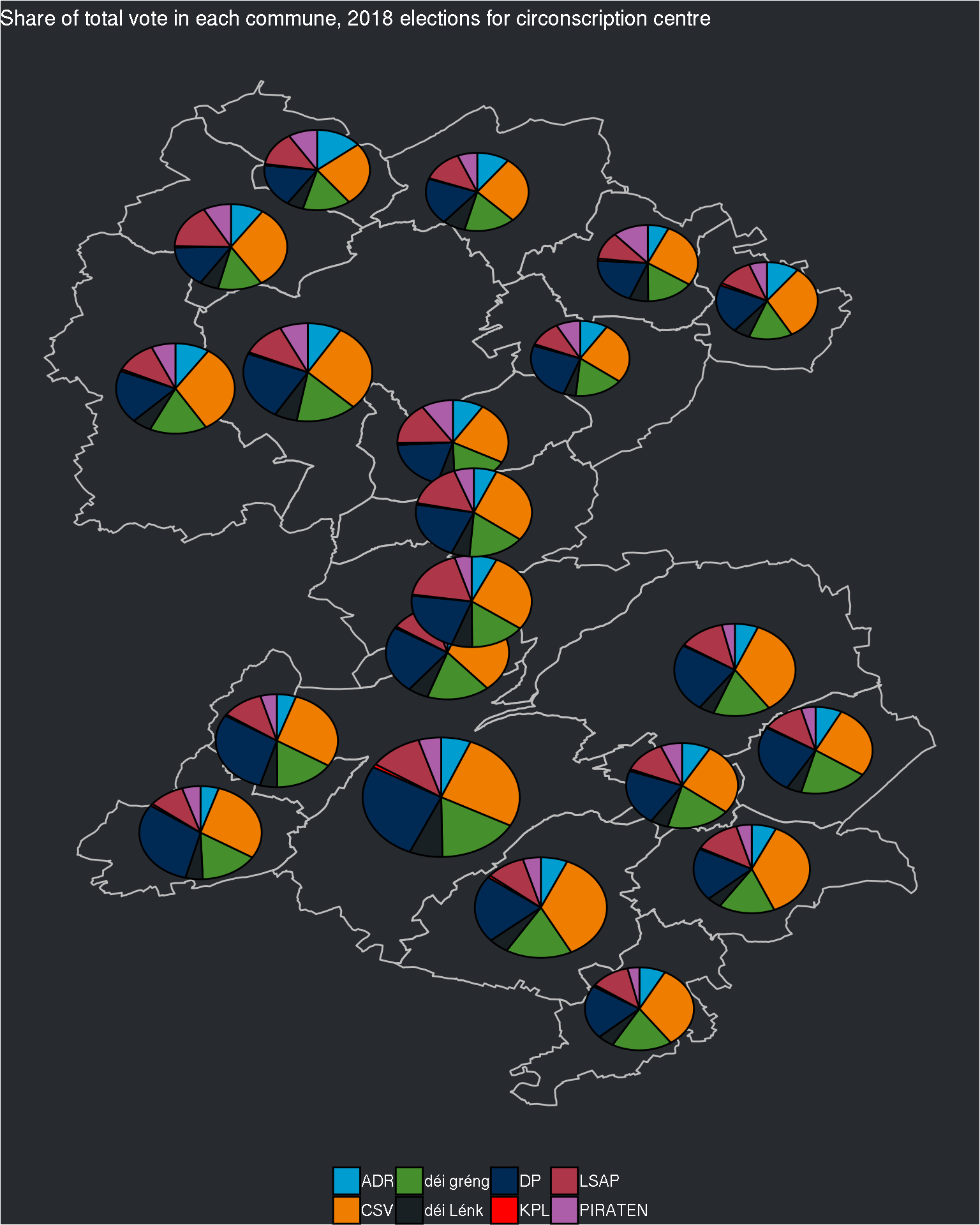``````##
## \$est``````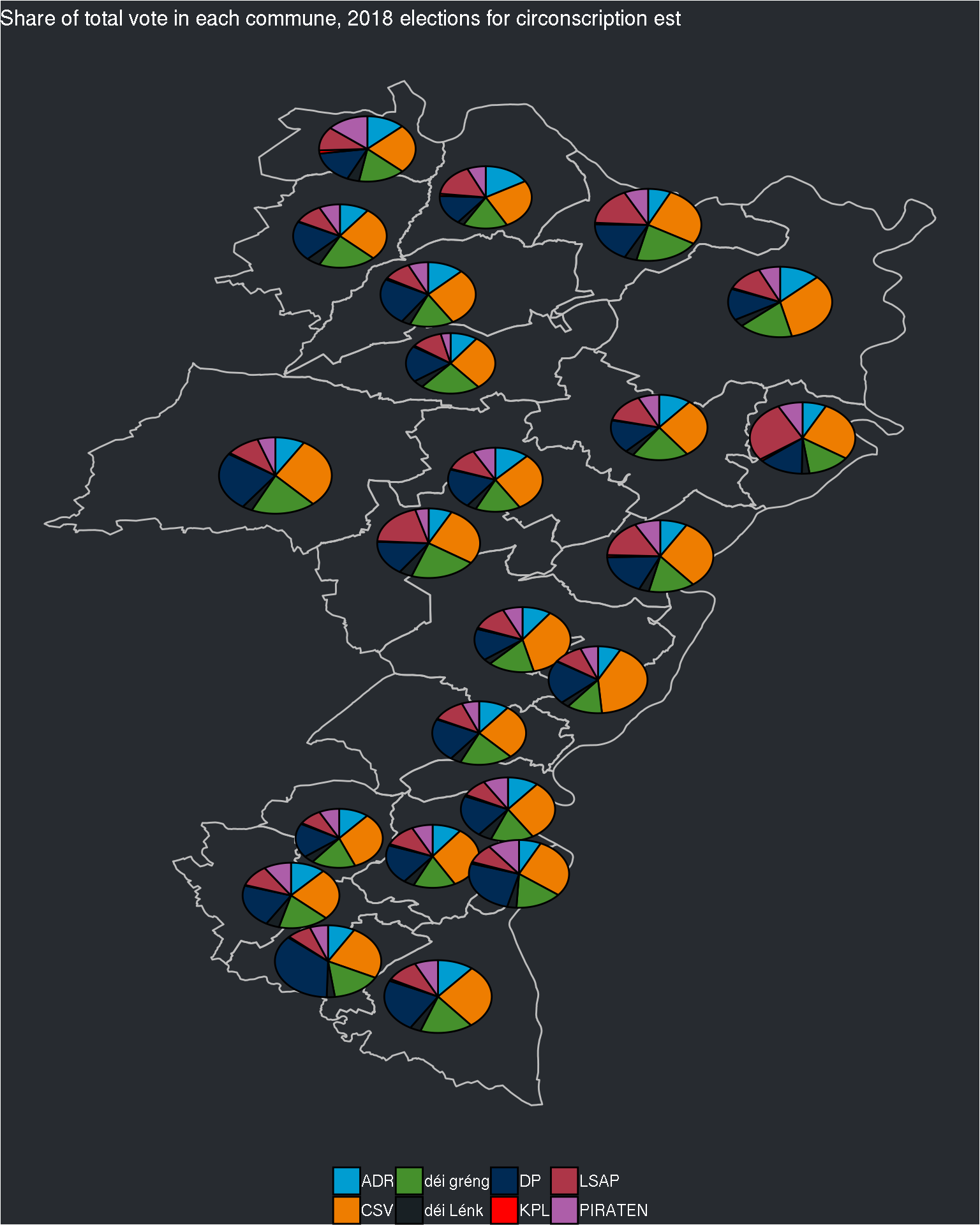``````##
## \$nord``````
``## Warning: Removed 16 rows containing non-finite values (stat_pie).``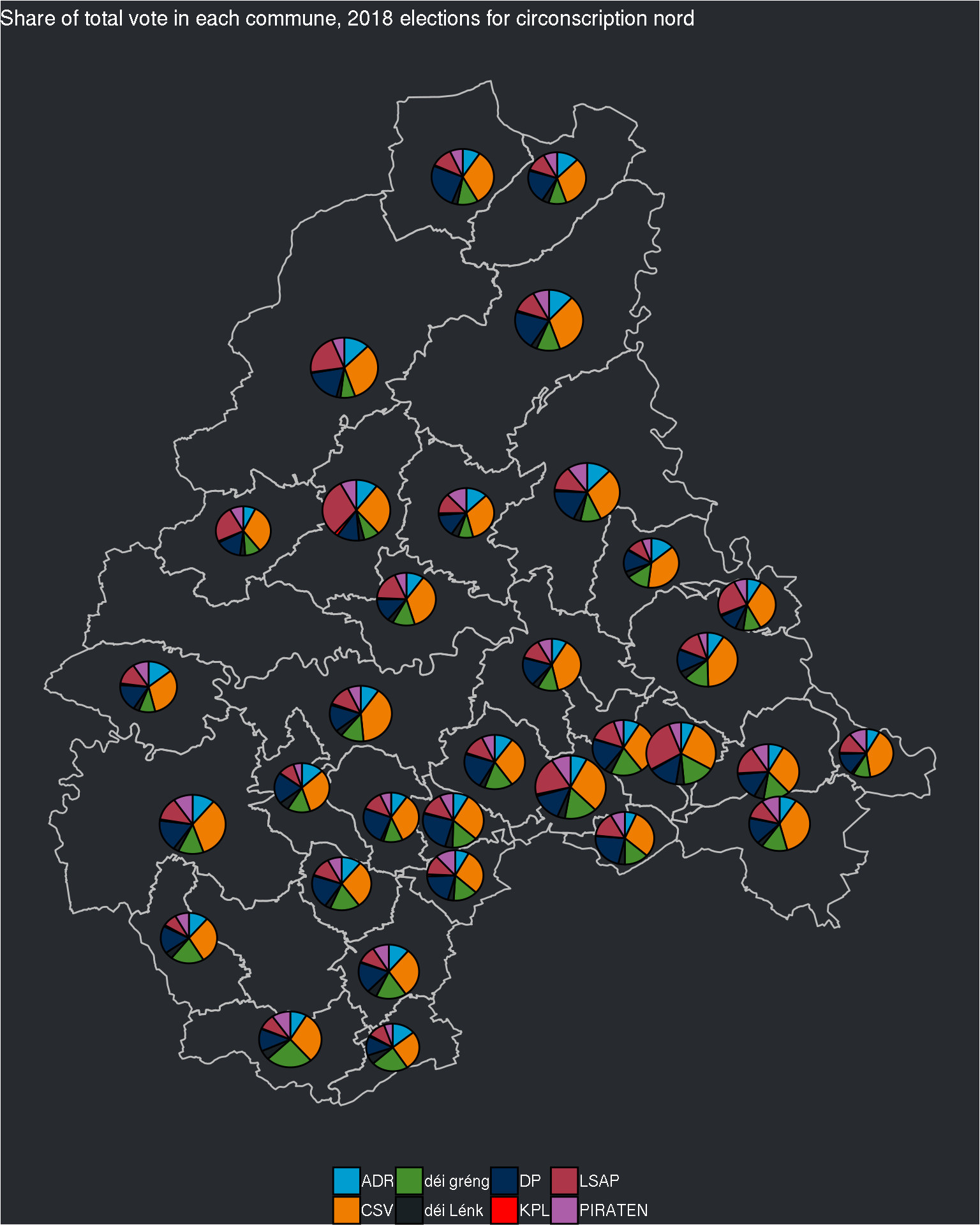``````##
## \$sud``````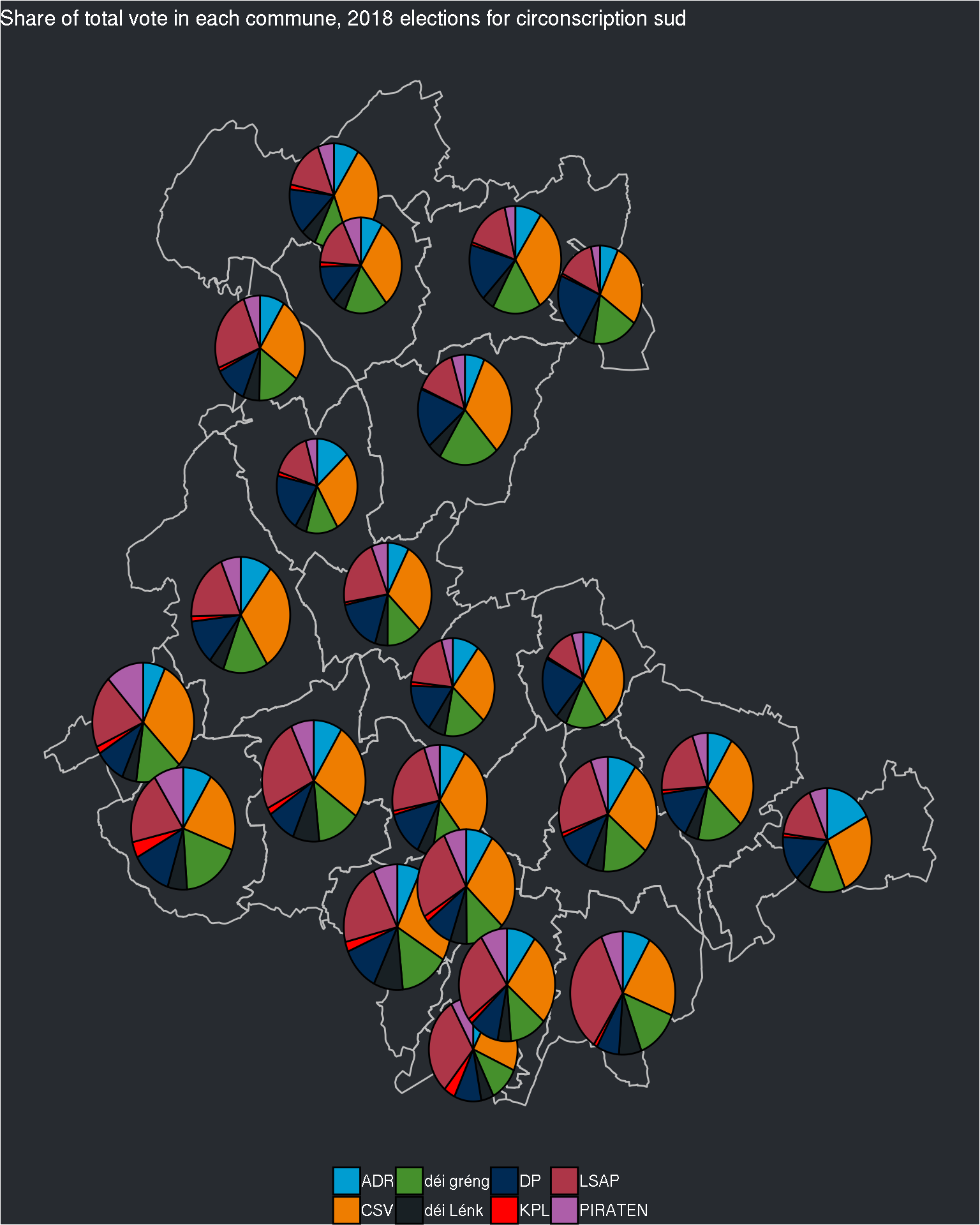This looks better now!

## Conclusion

Having data in a machine readable format is really important. The amount of code I had to write to go from the Excel Workbooks that contained the data to this plots is quite large, but if the data was in a machine readable format to start with, I could have focused on the plots immediately.

The good thing is that I got to practice my skills and discovered `{scatterpie}`!

Hope you enjoyed! If you found this blog post useful, you might want to follow me on twitter for blog post updates or buy me an espresso.

## Appendix

The following lines of code extract the data (from the 2013 elections) from the Excel Workbooks that can be found in Luxembourguish Open Data Portal.

I will not comment them, as they work in a similar way than in the previous blog post where I extracted the data from the 2018 elections. The only difference, is that the sheet with the national level data was totally different, so I did not extract it. The first reason is because I don’t need it for this blog post, the second is because I was lazy. For me, that’s two pretty good reasons not to do something. If you have a question concerning the code below, don’t hesitate to reach out though!

``````library("tidyverse")
library("tidyxl")
library("brotools")

path <- Sys.glob("content/blog/2013*xlsx")[-5]

elections_raw_2013 <- map(path, xlsx_cells) %>%
map(~filter(., sheet != "Sommaire"))

elections_sheets_2013 <- map(map(path, xlsx_sheet_names), ~`%-l%`(., "Sommaire"))

list_targets <- list("Centre" = seq(9, 32),
"Est" = seq(9, 18),
"Nord" = seq(9, 20),
"Sud" = seq(9, 34))

position_parties_national <- seq(1, 24, by = 3)

extract_party <- function(dataset, starting_col, target_rows){

almost_clean <- dataset %>%
filter(row %in% target_rows) %>%
filter(col %in% c(starting_col, starting_col + 1)) %>%
select(character, numeric) %>%
fill(numeric, .direction = "up") %>%
filter(!is.na(character))

party_name <- almost_clean\$character

almost_clean\$character <- "Pourcentage"

almost_clean\$party <- party_name

colnames(almost_clean) <- c("Variables", "Values", "Party")

almost_clean %>%
mutate(Year = 2013) %>%
select(Party, Year, Variables, Values)

}

# Treat one district

extract_district <- function(dataset, sheets, target_rows, position_parties_national){

list_data_districts <- map(sheets, ~filter(.data = dataset, sheet == .))

elections_districts_2013 <- map(.x = list_data_districts,
~map_df(position_parties_national, extract_party, dataset = .x, target_rows = target_rows))

map2(.y = elections_districts_2013, .x = sheets,
~mutate(.y, locality = .x, division = "Commune", Year = "2013")) %>%
bind_rows()
}

elections_2013 <- pmap_dfr(list(x = elections_raw_2013,
y = elections_sheets_2013,
z = list_targets),
function(x, y, z){
map_dfr(position_parties_national,
~extract_district(dataset = x, sheets = y, target_rows = z, position_parties_national = .))
})

# Correct districts
elections_2013 <- elections_2013 %>%
mutate(division = case_when(locality == "CENTRE" ~ "Electoral district",
locality == "EST" ~ "Electoral district",
locality == "NORD" ~ "Electoral district",
locality == "SUD" ~ "Electoral district",
TRUE ~ division))``````